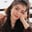Related Tags

cmath
phase

# What is cmath.phase(x) in Python?Fouzia Bashir

The phase(x) function can be used to get the phase of a complex number. It accepts integer, float, and complex type argument value x.

The output of cmath.phase(x) is equivalent to math.atan2 (x.imag, x.real), and the range lies between [-π, π].

Note: The cmath module in Python has multiple methods used for mathematical operations on complex numbers, just like the math module for floating-point numbers.

### Syntax

cmath.phase(x)


### Parameters

This method accepts the following arguments:

• x: The parameter value of which to find the phase.

### Return Value

It returns float value, representing the phase of a complex number x.

### Example

In this example, we discuss multiple types of argument values in cmath.phase(x).

• Integer: It will return a phase.
• Float: It will also return a phase.
• Complex: If x.imag is negative, then it does not matter (+ve answer). But when x.real is negative, then the result will be the same but the sign will be of x.imag. See line 8.
import cmath # include library
# Integer argument
print(cmath.phase(-90))
# floating point value as argument
print(cmath.phase(90.9))
# complex number as an argument value
# Complex(x.real, x.imag)
print(cmath.phase(complex(-1.0, -1.0)))
print(cmath.phase(complex(-1.0, 0.0)))

RELATED TAGS

cmath
phase

CONTRIBUTORFouzia Bashir
RELATED COURSES

View all Courses

Keep Exploring

Learn in-demand tech skills in half the time